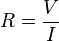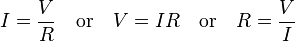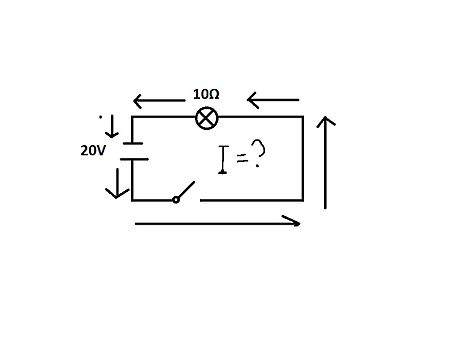# Ohm's law facts for kids

Kids Encyclopedia Facts

Ohm's law says that in an electrical circuit, the current passing through a resistor between two points, is related to the voltage difference between the two points, and inversely related to the electrical resistance between the two points. This relation is shown in the following formula:$R = \frac VI$

Where I is the current in amperes, V is the potential difference in volts, and R is a constant, measured in ohms, called the resistance.

Current is directly proportional to voltage loss through a resistor. That is, if the current doubles, then so does the voltage. To make a current flow through a resistance there must be a voltage across that resistance. Ohm's Law shows the relationship between the voltage (V), current (I) and resistance (R). It can be written in three ways:$I = \frac{V}{R} \quad \text{or}\quad V = IR \quad \text{or} \quad R = \frac{V}{I}$.

## Current, Voltage, and Resistance

### Voltage

Voltage is how much energy is between two points on a circuit. These two points have different charges, one is higher and the other is lower. The difference between these two points of the charge is how we measure the voltage.  The unit of “volt” is the name of the Italian physicist Alessandro Volta who created the first chemical battery.  The letter “V” represents voltage.

### Current

Current is how fast the charge is flowing. The higher the charge, the faster the current. Current has to do with electrons flowing in a circuit. Current measures how fast the electrons go. The unit of the current is “ampere,” and usually, a person writes it as “amps”. The letter “I” can represent as current.

### Resistance

Resistance is something to resist the flow of the charge, to make sure they not flow too fast and break the circuit. In a circuit, a light bulb can be a resistor. If electrons flow through the light bulb, then the light bulb will light up. If the resistance is high, then the light will be darker. The unit of resistance is “Ω”,  which is called omega, and pronounced “ohm”, it is the name of the inventor of Ohm’s law.

## How Current, Voltage, and Resistance are related

Current, Voltage, and Resistance are related, which is call it “Ohm’s law”. Ohm defines the unit of resistance of “1 Ohm” as the resistance between two points in a conductor where the application of 1 volt will push 1 ampere, or 6.241×10^18 electrons.

## Find all values in the circuitFor example, a scientist knows that the value of the voltage is 20V. He also knows the resistance, which is in the light bulb, is 10 Ω. He needs to find the other unknown variable, which is current. She can use the ohm’s law formula to solve it. With the two known variables, V(voltage) and R(resistance), the only variable left to find is I(current).

20V= 10Ω * I

I = 2A

In a problem, a scientist always gets enough information to solve the others values, the only thing a scientist has to memorize is the Ohm’s law formula. Then she uses it with what is given and solves for the unknown part. In the example above, the current is 2 amps.Ohm's law Facts for Kids. Kiddle Encyclopedia.